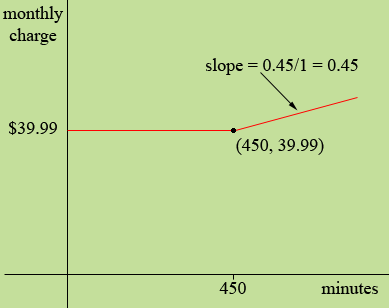SEARCH HOMEMath Central Quandaries & QueriesQuestion from John, a student: Hello, AT&T charges $39.99 for 450 minutes per month. It charges 45 cents per minute over the 450 minutes. How can I make a piecewise equation of this problem?Hi John, I would draw a graph with minutes on the horizontal axis and monthly charge on the vertical axis. From minutes = 0 to minutes = 450 the graph is a horizontal line at monthly charge =$39.99. At this point the line slopes upwards so that for each additional minute beyond 450 there is an added cost of \$0.45.My graph isn't to scale.

PennyMath Central is supported by the University of Regina and The Pacific Institute for the Mathematical Sciences.